# Math Practice Topic: Graphs to Linear Equations

 Description: Determine the linear equation for a graphed line. Adaptive Learning Progression: The y-intercept starts at 0, then the y-intercept changes, then the slopes become more complex. Start using MathScore for free

## Sample Levels (out of 7)

Write the equation in point slope format, y - y1 = m(x - x1), then convert into slope intercept form, y = mx + b.

 1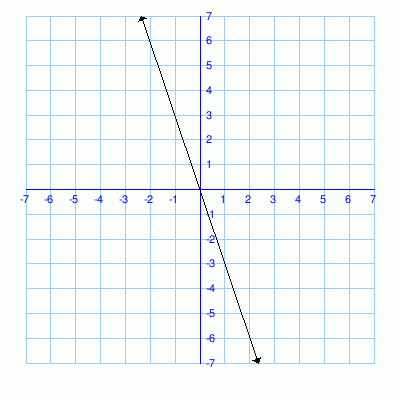Equation:

Write the equation in point slope format, y - y1 = m(x - x1), then convert into slope intercept form, y = mx + b.

 1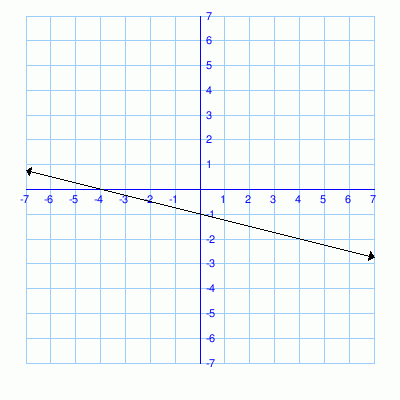Equation: 2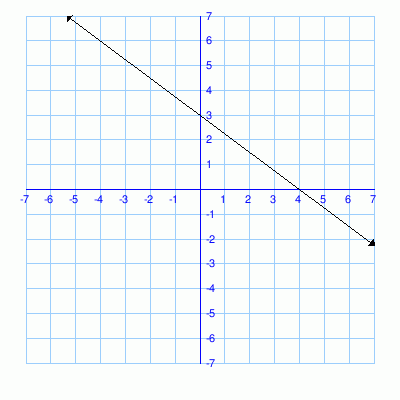Equation:

Write the equation in point slope format, y - y1 = m(x - x1), then convert into slope intercept form, y = mx + b.

 1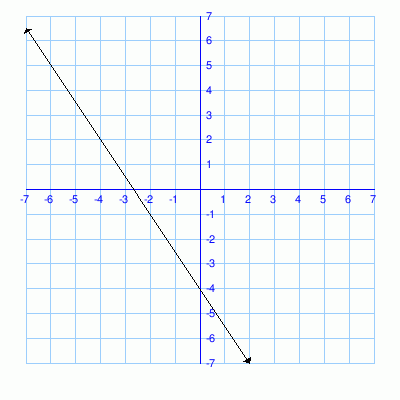Equation: 2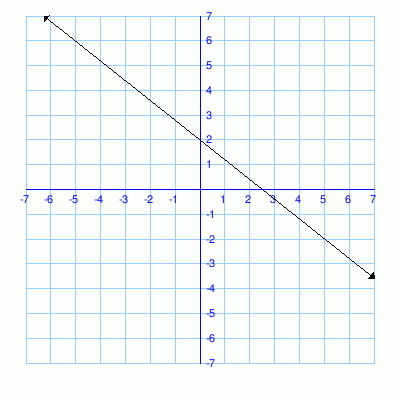Equation: 3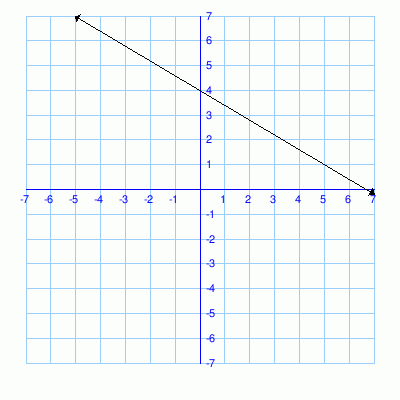Equation: